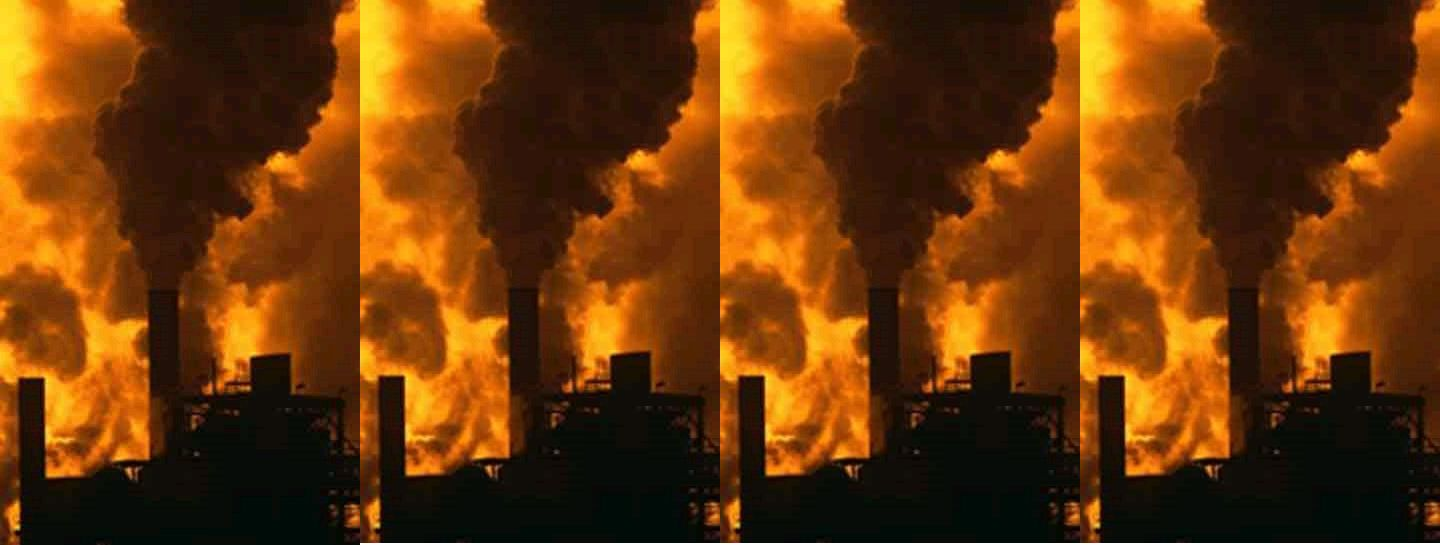# ZW Nitrogen Calculator

• In which I show that making synthetic nitrogen for the world takes the entire output of 120 power plants, twice as many if you include the natural gas used as a feedstock.

How much energy does it take to fix nitrogen using the chemically based Haber Process which requires natural gas as a raw material and then uses high pressure and temperature, both of which increase the energy budget by a large factor.

Some facts:
World production of synthetic ammonia is about 150 millions tons per year (Stanford Research Institute report).
The energy required by the Haber Process to make one ton of ammonia is about 40 billion joules, according to Shreve’s Chemical Process Industries ed. George Austin 5th Ed., McGraw-Hill (1984) p. 309.

• Converting: 40 billion joules per ton is about the same as 40 million BTU per ton or 20,000 BTU per pound.
• By coincidence, the heat energy of burning one pound of propane is also about 20,000 BTU.
• So one pound of propane is burned to produce one pound of ammonia.
• And 150 million tons of ammonia per year requires the burning of 150 million tons of propane.

How many power plants would we need to produce all of that energy from propane?

Multiplying by the 20,000 BTU from burning each pound of propane, we see that worldwide, synthetic ammonia requires:

• 150 million tons of propane times 2000 pounds per ton is 300 billion pounds of propane.  (1.5 x 10E8) x (2 x 10E3) = 3 x 10E11
• 300 billion pounds times 20,000 BTU from each pound yields 6000 trillion BTU from burning each year.  (3 x 10E11) x (2 x 10E4) = 6 x 10E15

Begin to convert to electrical units:

• Each BTU is about 1000 joules so 6000 trillion BTU per year is about 6 million trillion joules per year. (6 x 10E15) x (1 x 10E3) = 6 x 10E18
• Each year is about 40 million seconds so divide by 40 million to get the number of joules generated per second or 150 billion joules of energy per second. (6 x 10E18) / (4 x 10E7) = 1.5 x 10E11
• But one joule per second is the definition of one watt so the Haber Process worldwide uses 150 billion watts of power year round.
• A typical power plant produces one billion watts so the Haber Process requires the continual and dedicated services of 150 power plants year round.
• Since 20% of synthesized ammonia is used for non-agricultural purposes, multiply by 0.8 to get the number of power plants being used to produce agricultural ammonia. The result is 120 power plants.——————————————————————————–
Note:  Since the hydrogen in ammonia comes from additional propane, it is possible that this result should be approximately doubled, to 240 power plants. However, I am using the conservative result without this contribution.
——————————————————-
————————-